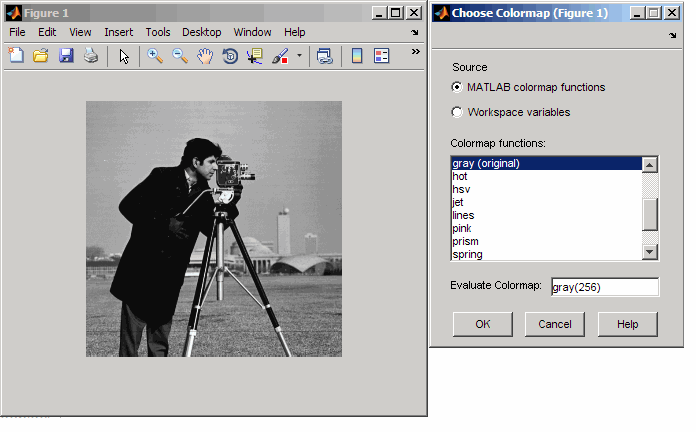# imcolormaptool

Choose Colormap tool

## Syntax

``imcolormaptool``
``imcolormaptool(hParent)``
``hTool = imcolormaptool(___)``

## Description

Use the `imcolormaptool` function to create a Choose Colormap tool. The Choose Colormap tool is an interactive colormap selection tool that allows you to change the colormap of a figure by selecting a colormap from a list of MATLAB® colormap functions or workspace variables, or by entering a custom MATLAB expression.

Choose Colormap Tool````imcolormaptool` opens the Choose Colormap tool for adjusting the colormap of the current figure.```

example

````imcolormaptool(hParent)` opens the Choose Colormap tool for adjusting the colormap of an image in parent figure or axes `hParent`. The figure or axes must contain a grayscale or an indexed image.```
````hTool = imcolormaptool(___)` returns the figure `hTool` containing the Choose Colormap tool.```

## Examples

collapse all

```h = figure; imshow("cameraman.tif") imcolormaptool(h)```
```fig = figure; ax1 = subplot(1,2,1); imshow("cameraman.tif") ax2 = subplot(1,2,2); imshow("canoe.tif") imcolormaptool(fig)```

## Input Arguments

collapse all

Graphics object containing the image, specified as a `Figure` or `Axes` object.

## Output Arguments

collapse all

Choose Colormap tool, returned as a `Figure` object.

## Version History

Introduced in R2009a